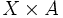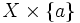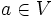# Tube lemma

(diff) ← Older revision | Latest revision (diff) | Newer revision → (diff)
Let$X$ be a compact space and$A$ any topological space. Consider$X \times A$ endowed with the product topology. Suppose$a \in A$ and$U$ is an open subset of$X \times A$ containing the entire slice$X \times \{ a \}$. Then, we can find an open subset$V$ of$A$ such that:$a \in V$, and$X \times V \subseteq A$